June 2019 was cooler than average (13.7°C versus an average of 14.4), wetter than average (104.20 mm versus an average total of 52.56) and less windy than average (a wind run of 2240 miles versus an average of 2408). The highest temperature in the month was 29.4°C, the highest temperature of 2019 so far, and the highest temperature since 31.0°C was recorded on July 26th 2018. This was the second highest June temperature recorded here, beaten only by the 29.5°C recorded on June 19th 2017. The minimum temperature was 7.0°C recorded at 05:03 on the 16th. The average temperature in [Read more]May 2019 was cooler than average (11.0°C versus an average of 11.5), drier than average (23.40 mm versus an average total of 44.13) and calmer than average (a wind run of 2132 miles versus an average of 2724). The highest temperature in the month was 21.3°C recorded at 16:30 on the 30th, 1.9°C below the mean for the month. The minimum temperature was 0.4°C recorded at 05:01 on the 5th, equalling the lowest May temperature set on May 5th 2012. The average temperature in the month was 11.0°C, 0.5°C below the mean. The average daily high temperature was 15.6°C, 0.2°C [Read more]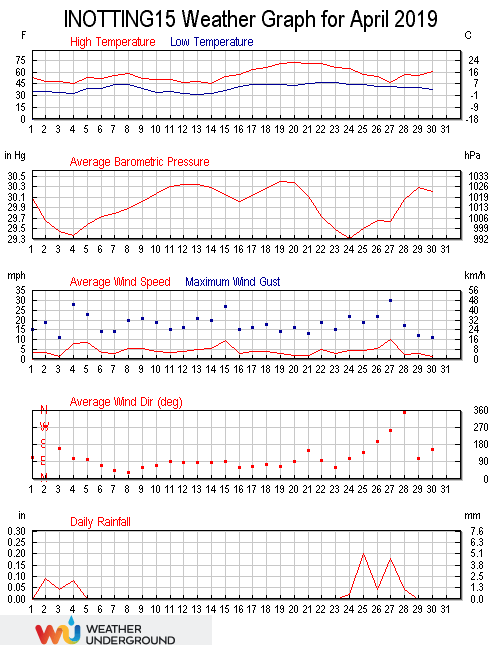April 2019 was warmer than average (8.6°C versus an average of 8.5°C), drier than average (17.60 mm versus an average total of 38.96) and windier than average (a wind run of 3094 miles versus an average of 2786). The month was very average with no records broken. The highest temperature in the month was at 17:04 on the 20th when the thermometer hit 22.2°C, the highest temperature of 2019 so far. The lowest temperature was -0.3°C at 06:15 on the 13th, the only time the temperature dropped below freezing in the month. The average temperature in the month was 8.6°C, [Read more]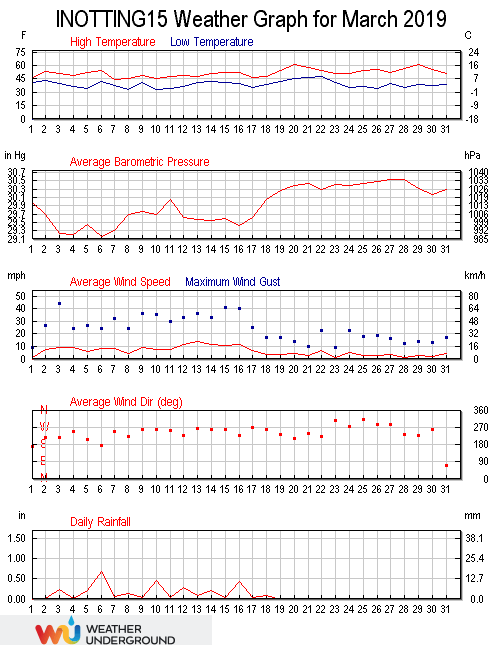March 2019 was warmer than average (7.4°C versus an average of 5.8°C), wetter than average (77.60 mm versus an average total of 40.56) and windier than average (a wind run of 4495 miles versus an average of 3112). March was a month of two distinct halves, the first 18 days being cold, damp and windy, with the final 13 days being milder and dry. The highest temperature in the month was 29th at 15:43 when 16.2°C was recorded. The lowest temperature was 0.6°C recorded at 07:00 on the 10th. This is the highest March minimum ever recorded here. The average [Read more]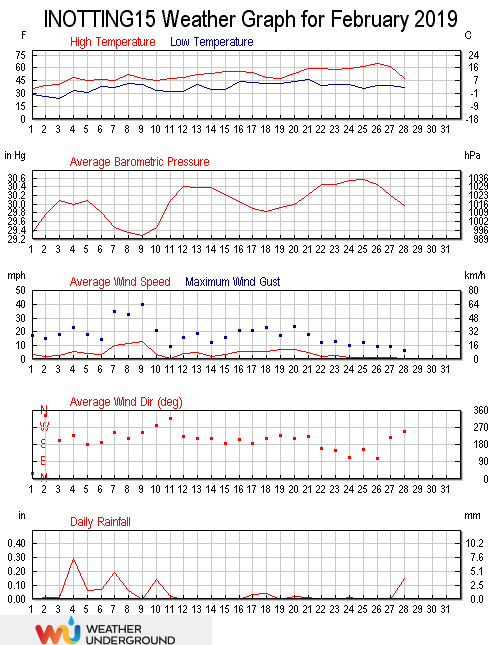February was warmer than average (6.7°C versus an average of 4.0°C), drier than average (28.00 mm versus an average total of 35.71) and calmer than average (a wind run of 2751 miles versus an average of 3108). An exceptional spell of warm weather in the second half of the month ensured that February 2019 was a record-breaker. The maximum temperature was 18.3°C at 14:44 on the 28th, beating the previous February high of 15.6°C set on February 23rd 2012. This was also the highest Winter temperature recorded here and also the February average of 6.7°C was the highest February average [Read more]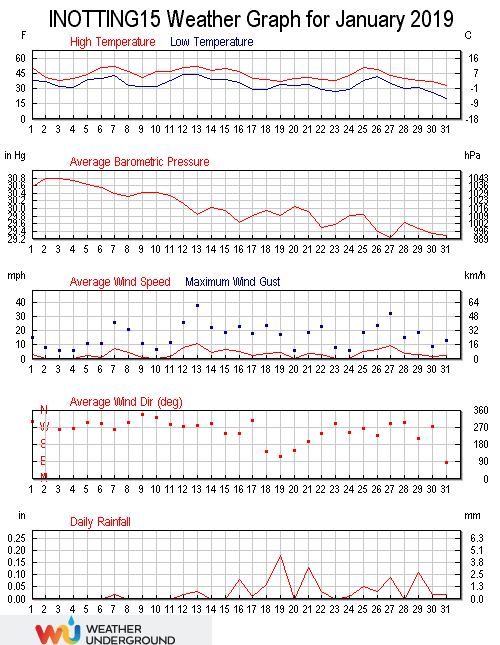January 2019 was average temperature at 3.8°C, drier than average (23.20 mm versus an average total of 44.42) and calmer than average (a wind run of 2667 miles versus an average of 3646). If it wasn’t for a record breaking cold spell in the final days of the month, January 2019 would have seen above average temperatures. A minimum temperature of -6.7°C recorded at 08:20 on the 31st was the lowest temperature since -7.2°C was recorded on February 11th 2012, and the lowest temperature ever recorded in January. The daytime maximum temperature of 0.4°C on the 31st was the lowest [Read more]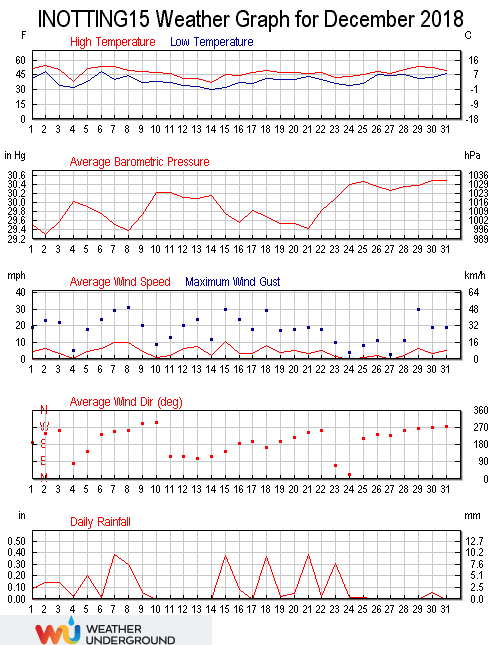December 2018 was warmer than average (6.3°C versus an average of 4.6), wetter than average (76.80 mm versus an average total of 47.91) and calmer than average (a wind run of 3206 miles versus an average of 3343). The maximum temperature in the month was 12.3°C recorded at 13:25 on the 2nd. The minimum temperature was -1.1°C recorded at 04:04 on the 14th, the lowest temperature of the winter season so far. The monthly average temperature was 6.3°C. The average daily high temperature was 8.6°C, with the average daily low temperature being 4.0°C. The warmest day was the 2nd with [Read more]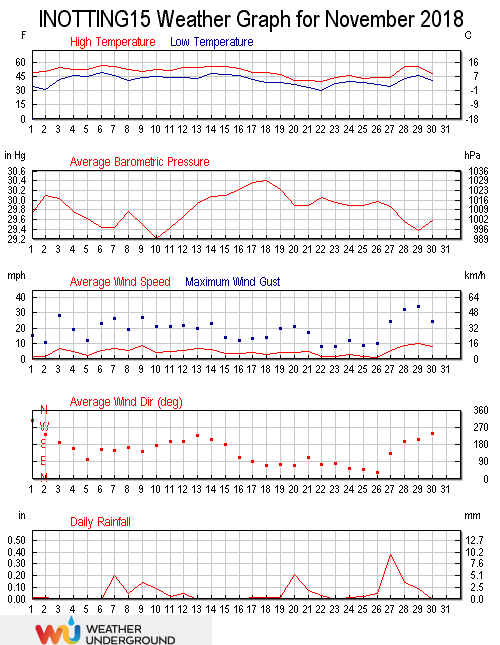November 2018 was warmer than average (7.6°C versus an average of 6.8), drier than average (40.40 mm versus an average total of 60.29) and windier than average (a wind run of 3325 miles versus an average of 2738). It was an unspectacular month with no records broken. The maximum temperature in the month was 14.1°C recorded at 14:28 on the 6th. The minimum temperature was -0.8°C recorded at 04:38 on the 22nd, with the monthly average being 7.6°C. The average daily high temperature was 10.1°C (0.6°C above the mean), with the average daily low temperature being 5.1°C (1.1°C above the [Read more]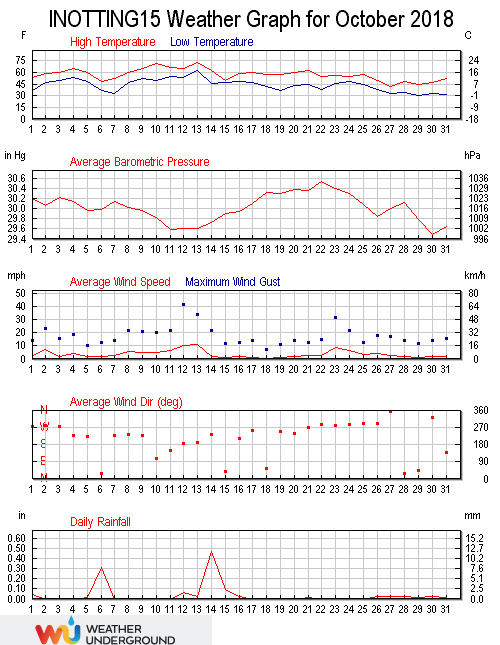October 2018 was cooler than average (10.1°C versus an average of 10.7), drier than average (27.80 mm versus an average total of 50.10) and windier than average (a wind run of 2740 miles versus an average of 2684). The maximum temperature recorded at 14:37 on the 13th was 22.7°C, the highest October temperature since 25.6°C was recorded on October 3rd 2011, and the fourth highest October temperature here. The minimum temperature, recorded at 07:01 on the 29th was -0.7°C. This was the lowest October temperature ever recorded here, beating the previous lowest of -0.4°C set on October 25th 2010. The [Read more]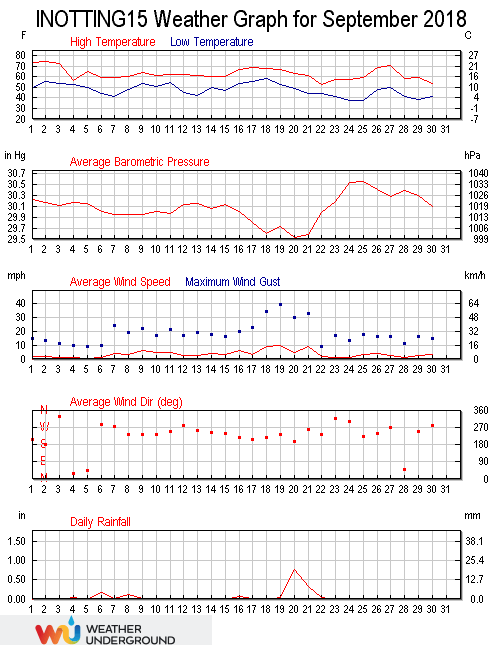September 2018 was cooler than average (13.0°C versus an average of 13.4), wetter than average (41.40 mm versus an average total of 36.68) and windier than average (a wind run of 2502 miles versus an average of 2162). September 2018 was cooler than average with the maximum being 23.9°C recorded at 15:29 on the 2nd, 0.1°C above the average high. The minimum temperature was 3.2°C, equalling the lowest ever September temperature recorded on September 22nd 2012. The maximum temperature on the 22nd, recorded at 14:02, was only 11.9°C making it the lowest daily maximum since May 2nd, when the maximum [Read more]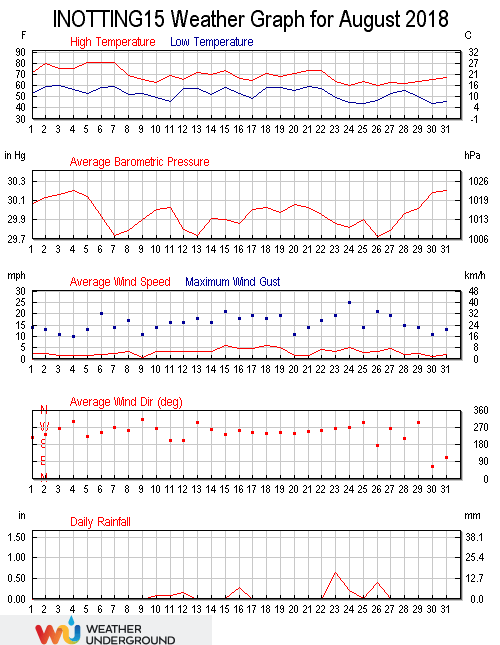August 2018 was warmer than average (16.4°C versus an average of 15.4), drier than average (49.20 mm versus an average total of 50.00) and calmer than average (a wind run of 2258 miles versus an average of 2273). Temperatures in August 2018 topped 20°C on 17 days. It wasn’t until the last week or so that the summer-long heatwave started to relent. The maximum temperature in the month was 27.7°C, recorded at 17:06 on the 5th. The minimum temperature was 6.9°C recorded at 06:28 on the 30th, with the monthly average being 16.4°C, beating the previous August record set in [Read more]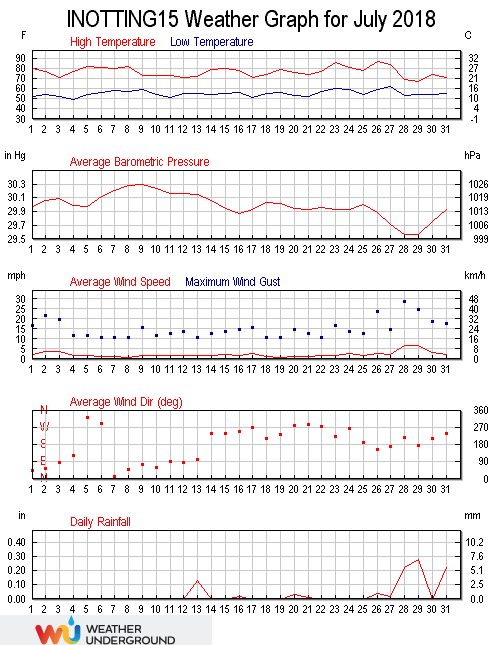July was warmer than average (18.9°C versus an average of 16.5), drier than average (24.40 mm versus an average total of 47.33) and calmer than average (a wind run of 1700 miles versus an average of 2085). July 2018 was a very warm month with temperatures topping 20°C on all but the 29th, when the maximum only reached 19.5°C, ending a run of 35 consecutive days of above 20°C maximum temperatures, easily a record for here. The maximum temperature in the month was 31.0°C recorded at 17:35 on the 26th. This was only the fourth time that a temperature in [Read more]